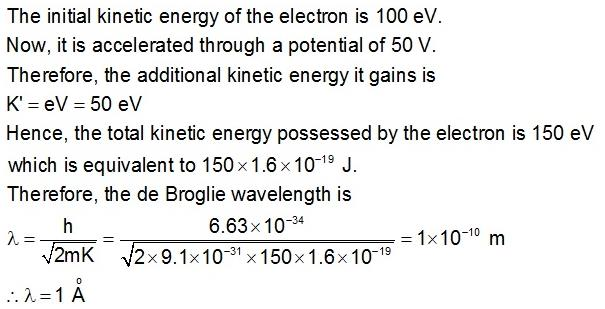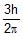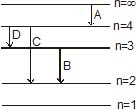Courses

# Test: Dual Nature of Radiation and Matter- 2

## 30 Questions MCQ Test Physics For JEE | Test: Dual Nature of Radiation and Matter- 2

Description
This mock test of Test: Dual Nature of Radiation and Matter- 2 for Class 12 helps you for every Class 12 entrance exam. This contains 30 Multiple Choice Questions for Class 12 Test: Dual Nature of Radiation and Matter- 2 (mcq) to study with solutions a complete question bank. The solved questions answers in this Test: Dual Nature of Radiation and Matter- 2 quiz give you a good mix of easy questions and tough questions. Class 12 students definitely take this Test: Dual Nature of Radiation and Matter- 2 exercise for a better result in the exam. You can find other Test: Dual Nature of Radiation and Matter- 2 extra questions, long questions & short questions for Class 12 on EduRev as well by searching above.
QUESTION: 1

### Let nr and nb be respectively the number of photons emitted by a red bulb and a blue bulb of equal power in a given time.

Solution:

Since, Pr​=Pb​
r for red and b for blue.
Pr​=Pb​
or, nr​× (hc/λr)  ​=nb​× (hc​/λb)​
or,  ​(nr/nb)​​= λr​​/λb
Since, the wavelength of red bulb is greater than the wavelength of blue bulb.
or,  nr​>nb

QUESTION: 2

### 10-3 W of 5000 Å light is directed on a photoelectric cell. If the current in the cell is 0.16 mA, the percentage of incident photons which produce photoelectrons, is

Solution:

Current is 0.16×10−6 Amp it means 0.16×10−6 Coulomb charge is flowing per second
So, n=0.16×10−6C /1.6×10−19C ​=1012 electrons are generated per second
Now we notice that one photon has energy E, E=hc/λ=​=(6.62×10−34Js×3×108ms-1)/(5000×10−10m) ​=3.972×10−19Joule
So, number of photon in 10−3W will be N=10−3/3.972×10−19 ​=0.25×1016 this is number of photons incident per second
So required percentage is (n/N)×100=1014/(0.25×1016) ​=0.04%

QUESTION: 3

### If the frequency of light in a photoelectric experiment is doubled, the stopping potential will

Solution:

The maximum kinetic energy for the photoelectrons is
Emax​=hν−ϕ
where, ν is the frequency of incident light and ϕ is photoelectric work function of metal.
eV0​=hν−ϕ ...................(1)
where, V0​ is the stopping potential and e is the electronic charge.
When, the frequency of light in a photoelectric experiment is doubled,
eV0′​=2hν−ϕ
eV0′​=2[hν−(ϕ/2​)].........................(2)
From the above two equations we can say that the K.E. in (2) is more than double of K.E in (1). Hence, when the frequency of light in a photoelectric experiment is doubled, the stopping potential becomes more than double.
So, the answer is option (C).

QUESTION: 4

The stopping potential for the photo electrons emitted from a metal surface of work function 1.7eV is 10.4 V. Identify the energy levels corresponding to the transitions in hydrogen atom which will result in emission of wavelength equal to that of incident radiation for the above photoelectric effect

Solution:

As we know that the stopping potential of the photoelectron is equal to the maximum kinetic energy of the photoelectron,
KEmax​=10.4V
Now, in photoelectric effect,
Energy of incident radiation (Ein​) = work function + K.Emax​
⇒ Ein​=1.7+10.4
⇒ Ein​=12.1eV
Now, for 0 hydrogen atom,
Energy of first energy level, E1​=−13.6eV
Energy of second energy level, E2​=−3.4eV
Energy of third energy level, E3​=−1.5eV
Hence, a transition from third to first energy level will result in emission of radiation of energy = E3​−E1​=12.1eV which is same as the energy of incident radiation of above photoelectric effect.
Thus, correct answer is n=3 to 1

QUESTION: 5

When a photon of light collides with a metal surface, number of electrons, (if any) coming out is

Solution:

When photon strikes with the electron it completely transfers it’s energy to the electron as during photoelectric experiment the threshold frequency required is used by the electron to eject from the atom which is also called the work function and the remaining energy in electron is kinetic energy which can be measured. Now (before collision) since photon is a particle it must have mass and thus it has energy equivalent to E=mc2 .
After collision when it completely transfers it’s energy to electron thus E=0
And therefore 0=mc2 thus i guess photon vanishes.

QUESTION: 6

A point source of light is used in photoelectric effect. If the source is removed farther from the emitting metal, the stopping potential

Solution:

When the source is moved away from the emitter, intensity of the incident radiation decreases but frequency remains the same so there will be no change in the stopping potential. Thus, it remains constant.

QUESTION: 7

A point source causes photoelectric effect from a small metal plate. Which of the following curves may represent the saturation photocurrent as a function of the distance between the source and the metal?

Solution:

Saturation current is the maximum current possible and it will be directly proportional to the number of number of electrons falling on collector plate per second which depend on number of photons
incident on the cathode as one photon contribute in one electron and the number of photons is actually
proportional to intensity which varies
As intensity I∝1/r2​, where r is the distance
So the correct graph will be decreasing with power2 of distance and it will be rapidly decreasing with a higher value of r.

QUESTION: 8

The maximum kinetic energy of photoelectrons emitted from a surface when photons of energy 6 eV fall on it is 4eV. The stopping potential is Volts is

Solution:

Given, the maximum kinetic energy: Kmax​=4eV
If V0​ be the stopping potential, then Kmax​=eV0​
⇒eV0​=4eV
⇒V0​=4V

QUESTION: 9

Radiation of two photon energies twice and five times the work function of metal are incident sucessively on the metal surface. The ratio of the maximum velocity of photoelectrons emitted is the two cases will be

Solution:

As,
Maximum K.E= incident photon energy − work function
(½)​mV12​=2W−W=W⟶(1)
and
Ve​mV22​=5W−W=4W⟶(2)
from (1) and (2)
V1/V2​ ​​=1/2​
V1​:V2​=1:2

QUESTION: 10

Cut off potentials for a metal in photoelectric effect for light of wavelength l1, l2 and l3 is found to be V1, V2 and V3 volts if V1, V2 and V3 are in Arithmetic Progression and l1, l2 and l3 will be

Solution:

We know that,
eV=(hc/λ)-w
V=(hc/eλ)-(w/e)
Arithmetic progression =>V2=(V1+V2)/2
Now,
(hc/eλ2)-w/e=1/2[(hc/eλ1)-(w/e) +(hc/eλ3) -(w/e)]
=>1/ λ2=1/2[(1/ λ1)+(1/λ3)]
=>2/ λ2=1/ λ1 + 1/λ3
Hence the correct answer is harmonic Progression.

QUESTION: 11

Photons with energy 5eV are incident on a cathode C, on a photoelectric cell. The maximum energy of the emitted photoelectrons is 2eV. When photons of energy 6eV are incident on C, no photoelectrons will reach the anode A if the stopping potential of A relative to C is

Solution:

When 5eV is incident the kinetic energy is 2eV it simply means the work function is W=5eV−2eV=3eV
Similarly, when 6eV is incident the kinetic energy should be   6eV−W=6eV−3eV=3eV
it simply means to stop them we need a negative potential at anode equal to 3eV/e​=3V
So, the answer is −3V i.e. option B is correct.

QUESTION: 12

By increasing the intensity of incident light keeping frequency (v > v0) fixed on the surface of metal

Solution:

The number of photoelectrons emitted per second from a photosensitive plate is directly proportional to the intensity of the incident radiation. ... For the same frequency of light and increased intensity, the saturation current is found to increase, but the cut-off potential is found to remain constant.
The number of electrons also changes because of the probability that each photon results in an emitted electron are a function of photon energy. If the intensity of the incident radiation of a given frequency is increased, there is no effect on the kinetic energy of each photo electron.

QUESTION: 13

In a photoelectric experiment, electrons are ejected from metals X and Y by light of intensity I and frequency f. The potential difference V required to stop the electrons is measured for various frequencies. If Y has a greater work function than X ; which one of the following graphs best illustrates the expected results ?

Solution:

First, the gradient of the graph cannot change (always = h/e ), so answers are A or D.
If Y has a greater work function than X, then the graph for Y should have a more negative y-intercept. therefore figure in option A depicts the concept.

QUESTION: 14

A image of the sun of formed by a lens of focal-length of 30 cm on the metal surface of a photo-electric cell and a photo-electrci current is produced. The lens forming the image is then replaced by another of the same diameter but of focal length 15 cm. The photo-electric current in this case is

Solution:

Lenses of the same diameter collect equal amounts of light.
Intensity is the measure of amount of light collected, hence the intensity remains the same.
Intensity is measured by the photoelectric current. So the photoelectric current would also remain the same.

QUESTION: 15

A proton and an electron are accelerated by same potential difference have de-Broglie wavelength lp and le.

Solution:

Charge present on both particles are same
∵λ= h​/mqV so q1​=q2
λe​​p​ = √(mp​v​​/me​v) =>1
∵mp​>>me​
∴λe​p​.
Option C is the correct answer.

QUESTION: 16

An electron with initial kinetic energy of 100eV is acceleration through a potential difference of 50V. Now the de-Broglie wavelength of electron becomes.

Solution:QUESTION: 17

If h is Planck's constant is SI system, the momentum of a photon of wavelength 0.01 Å is

Solution:

Momentum of photo, p=E/c​=hν/c ​where E is the energy of a photon and c is the velocity of light.
∴ p= hc/cλ ​    [∵ν=λc​]
p=h/λ​=h/(0.01×10−10)​=1012h

QUESTION: 18

The angular momentum of an electron in the hydrogen atom is. Here h is Planck's constant. The kinetic energy of this electron is

Solution:

Angular momentum,
L=nh/2π​=3h​/2π (given)
also n=3
∴  E=(−1.36​/ n2)eV
E=−13.6/(3)2​ eV
E=−13.6/9 ​eV
E=−1.57eV

QUESTION: 19

Consider the following electronic energy level diagram of H-atom : Photons associated with shortest and longest wavelengths would be emitted from the atom by the transitions labelled.Solution:

ΔE=k[(1/n22)-1/n12]=hc/λ
If transition from n-4->n=2
ΔE-->Maximum energy.
Wavelength λ will be the shortest for C.
If transition occurs from n=4--->n=3
Then, ΔE--->Minimum
Wavelength λ will be longest for D.

QUESTION: 20

If the electron in a hydrogen atom were in the energy level with n = 3, how much energy in joule would be required to ionise the atom ? (Ionisation energy of H-atom is 2.18 × 10-18 J)

Solution:

Energy of e− in nth shell =−13.6 (τ2​ /n2)
For H−atom with n=3→E=−13.6×(1/9)​
⇒E=1/9​ (Energy of e− in first shell)
⇒E=(1/9)​×2.18×10−18)
⇒E=2.42×10−19J(Ionisation Energy= Energy of e in first shell)

QUESTION: 21

In a hydrogen atom, the electron is in nth excited state. It may come down to second excited state by emitting ten different wavelengths. What is the value of n

Solution:

Total no. of levels =n−1
n→2
Put n′=n−1 in the formula attached
(n−1)(n−2)/2​=10
(n−1)(n−2)=20
⇒n=6

QUESTION: 22

Difference between nth and (n + 1)th Bohr's radius of `H' atom is equal to it's (n _ 1)th Bohr's radius. The value of n is

Solution:

We know that rn​∝n2
Given: rn+1​−rn​=rn−1
∴(n+1)2−n2=(n−1)2
n=4

QUESTION: 23

The electron in a hydrogen atom makes transition from M shell to L. The ratio of magnitudes of initial to final centripetal acceleration of the electron is

Solution:

According to Bohr's atomic theory,
radius of nth orbit, r ∝ n2/Z
velocity of electron in nth orbit, v ∝ Z/n
then acceleration of electron in nth orbit, a = v2/r
= (Z/n)2/(n2/Z)
= Z3/n4
so acceleration, a ∝ 1/n4
now for m shell, n = 3
for l shell, n = 2
now initial acceleration/final acceleration = a₁/a₂
= (n2/n1)4 = (2/3)4 = 16/81
Therefore the ratio of initial to final acceleration is 16:81.

QUESTION: 24

The electron in a hydrogen atom makes a transition n1 → n2 whose n1 and n2 are the principal quantum numbers of the two states. Assume the Bohr model to be valid. The frequency of orbital motion of the electron in the initial state is 1/27 of that in the final state. The possible values of n1 and n2 are

Solution:

frequency∝ z2​/n3
⇒f=kz2/n3​
∴f1​=(1/27) f2
⇒​kz12/n13 ​​=(1/27) (kz22/n23​)   ​​(z1​=z2​=1)
⇒k/n13​​=(1/27) (k/n23)
⇒n1​=3n2​
∴n1​=3 and n2​=1

QUESTION: 25

The radius of Bohr's first orbit is a0. The electron in nth orbit has a radius

Solution:

rn​= ϵ0​n2h2​/ πmZe2
Wherein
=rn​∝ n2​ /Z
= ϵ0​h2/πme2 ​=0.529
r=(n2​/Z)a0
For Z=1 r=n2a0

QUESTION: 26

The ionisation potential of hydrogen atom is 13.6 volt. The energy required to remove an electron from the second orbit of hydrogen is :

Solution:

Ionization energy-
Eion=E-En
=13.6 (Z2/n2)
- wherein
Energy required to move an electron from ground state to
n=∞
Energy required to remove electron from 2nd bohr orbit is
△E=E0 . [1/{22-(1/∞)}]=E0/4=(13.6/4) eV
△E=3.4eV

QUESTION: 27

In a sample of hydrogen like atoms all of which are in ground state, a photon beam containing photons of various energies is passed. In absorption spectrum, five dark lines are observed. The number of bright lines in the emission spectrum will be (Assume that all transitions take place)

Solution:

Absorption spectrum is observed when a photon is observed and a electron
Jumps to a higher state. Controversy emission spectrum is observed when
electron comes to lower state emitting a photon
On considering all possible transition states, thus no. of observation
and emission lines will be the same i.e. 5.

QUESTION: 28

When a hydrogen atom, initially at rest emits, a photon resulting in transition n = 5 → n = 1, its recoil speed is about

Solution:

Given,
n1​=1
n2​=5
m=1.6×10−27kg
Momentum, P=mv=h​/λ
recoil speed, v= h​/mλ. . . . . . . . .(1)
we know that,
1/λ​=R[(1/n12)​​−(1/n22​​)]
1/λ ​=1.097×107[(1/12​)−(1/52​)]
λ=9.48×10−8m
From equation (1),
recoil speed, v=6.67×10−34​/1.6×10−27×9.48×10−8 =4.2m/s
The correct option is C.

QUESTION: 29

Consider the spectral line resulting from the transition n = 2 → n = 1 in the atoms and ions given below. The shortest wavelength is produced by :

Solution:

Wavelength is calculated as
1/λ=Rz2(1/nf2​​=1/ni2​​)
∴λ∝1/z2
For hydrogen atom, z=1
For Deuterium atom, z=2
For singly ionized helium, z=2
For doubly ionized lithium, z=3
Thus, z is maximum for lithium and so λ is minimum.
Hence the correct answer is option D.

QUESTION: 30

In an atom, two electrons move around the nucleus in circular orbits of radii R and 4R. The ratio of the time taken by them to complete one revolution is : (neglect electric interaction)

Solution:

Time period, T∝(R)3/2
∴ T1/T2​ ​​= (​R1/R2 ​)3/2= (1/4​)3/2=1/8
Thus, the ratio of time period is 1:8